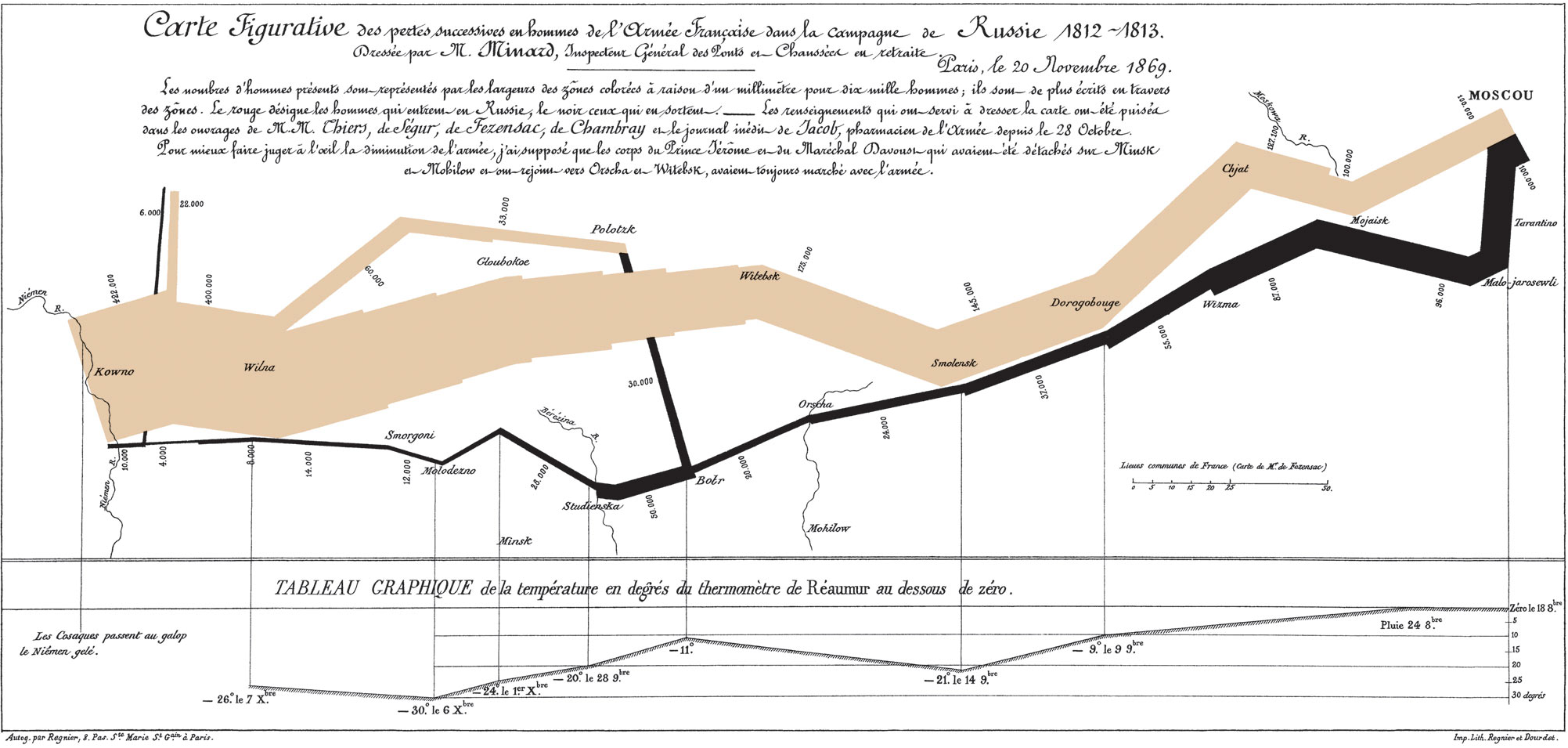# Notes for A Layered Grammar of Graphics

## 2018/04/02# A data visualization example from A LAYERED GRAMMAR OF GRAPHICS

library(ggplot2)

troops <- read.table("https://github.com/XUKEREN/KERENXU/files/1866822/minard-troops.txt", header=T)
cities <- read.table("https://github.com/XUKEREN/KERENXU/files/1866821/minard-cities.txt", header=T)

# Set the object size as the number of survivors

plot_troops <- ggplot(troops, aes(long, lat)) +
geom_path(aes(size = survivors, colour = direction, group = group))
plot_troops# Add the cities on the plot according to their coordinates

plot_both <- plot_troops +
geom_text(aes(label = city), size = 4, data = cities)
plot_bothplot_polished <- plot_both +
scale_size(range = c(1, 12),
breaks = c(1, 2, 3) * 10^5, labels =(c(1, 2, 3) * 10^5)) +
scale_colour_manual(values = c("grey50","red")) +
xlab(NULL) +
ylab(NULL)
plot_polished# Save plots in pdf

ggsave(plot_troops, file = "minard-troops.pdf", width=12, height=3)
ggsave(plot_both, file = "minard-both.pdf", width=12, height=3)
ggsave(plot_polished, file = "minard-polished.pdf", width=12, height=3)

# Another example from the ‘A layered Grammar of Graphics’

library(ggplot2)

## continuous variable mapped to size and colour, discrete variable mapped to shape and colour.

### Examples of axes and grid lines for three coordinate systems:

#### illustrates the difficulties associated with non-Cartesian

x1 <- c(1,10)
y1 <- c(1, 5)
p <- qplot(x1, y1, geom="blank", xlab=NULL, ylab=NULL) + theme_bw()
pp + coord_trans(y="log10")p + coord_polar()### A bullseye chart is the polar equivalent of the spineplot: in the pie chart, categories have equal radius and variable angle; in the radial chart, categories have equal angle and variable radius.

bars <-  qplot(clarity, data=diamonds, geom="blank", fill=clarity) +
scale_fill_brewer(palette="YlGnBu")
stack <- bars + aes(x = factor(1)) + scale_x_discrete(labels = "")
stack + geom_bar(width = 1) + coord_polar(theta="y")stack + geom_bar(width = 1) + coord_polar()### The Coxcomb plot is a bar chart in polar coordinates.

bars + geom_bar()bars + geom_bar(width = 1) + coord_polar()